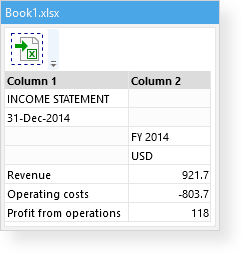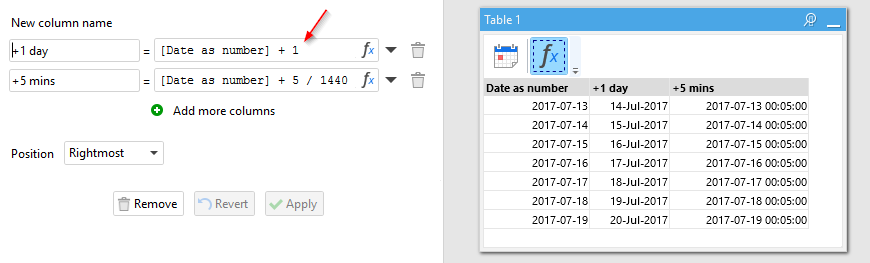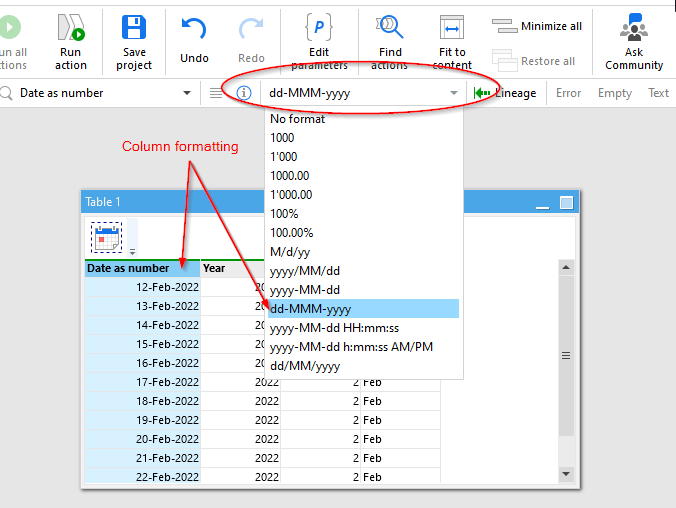# Type system and expressions

In EasyMorph imported and calculated values can be of one of the following types:

• Number
• Text
• Boolean
• Error
• Empty

A table column in EasyMorph can contain a mix of values of any type. For instance, text values and numbers can be mixed in the same column.You can guess a value's type by looking at its alignment in the datagrid. In tables, text is always aligned left, while numbers are always aligned right. That's how you can distinguish if a number is indeed a number, and not a text value consisting of digits. Booleans are either TRUE or FALSE, aligned to the middle. Errors are always red. Empty values have a very light gray background.

### Text

Text values in EasyMorph are text strings in the (UTF-8 encoding). To concatenate text strings, use the ampersand (&). In expressions, text constants should be wrapped either in single or in double quotes. For instance:

`'Easy' & "Morph"`
`replace('03-2016', '-', '/')`

To deal with single quotes, use double quotes and vice versa.

`replace('"ABC"', '"', "'")`

### Numbers

Numbers are either integer or decimal numbers with a fixed decimal point. Floating point numbers and numbers in the exponential notation are not supported. The effective range for numbers in EasyMorph is approximately (-7.9 x 1028 to 7.9 x 1028) / (100 to 28) which is 28-29 significant digits with or without decimal point between them.

### Dates

Notice there is no dedicated type for dates in EasyMorph. Instead, as in Excel, dates are numbers that are formatted as dates. Such a number date represents the number of days since January 1st, 1900. For instance, 42680 corresponds to November 6th, 2016 because there are 42680 days from January 1st, 1900 to that date.

Dates in expressions can be specified using date literals. A date literal has the format #yyyy-MM-dd. For example:

`#2016-11-06`

is the same as 42680 and corresponds to November 6th, 2016. Here is an example of date literals in a condition:

`[Date] >= #2016-10-01 and [Date] <= #2016-12-31`

Because dates are numbers, there is no need to use a special function for adding days. To get the next day just add 1. However, there are special functions for adding months — addmonth(), and years — addyears(). These functions deal correctly with leap years.### Number/date formatting

Since dates in EasyMorph are just numbers, column formatting can help distinguish dates. To change column formatting, select a column, and then choose a format from the column bar above the workspace (see the screenshot below).Note that formatting doesn't affect values. In other words, if you change the column formatting, the column values don't change. They are just displayed differently in the datagrid.

By default, numeric values are not formatted. Although, for new columns, EasyMorph can attempt to auto-detect dates and automatically set column formatting to a date format. In some cases, the auto-detection doesn't work correctly. For instance, a range of US zip codes can be mistakenly detected as dates. If you see an incorrectly detected column format, don't panic. Just select the necessary format from the column bar and save the project. EasyMorph will remember your preference for that column.

### Boolean

Boolean values can only be either TRUE or FALSE. Most often they are a result of a comparison or logical function, and are frequently used in conditions (more on that below).

### Empty values

If a table cell has no value — it's called an empty value. Empty values are somewhat similar to nulls in relational databases, but not exactly. For instance, an empty value always equals another empty value, and is equal to an empty string in expressions. All these comparisons return TRUE:

`<empty value> = <empty value>`
`<empty value>  = ''`
`<empty value>  = ""`

You can check if a column value is empty using the isempty() function. The length of an empty value is always zero. To create an empty value, use the empty() function. For example:

`if( [Column 1]='AAA', 'Triple A', empty() )`

Hint: In the example above, the if() function has the following syntax `if(condition, result_if_true, result_if_false)`. It is similar to the same function in Excel.

In tables, empty values have a very light gray background (possibly unnoticeable on some monitors). That's how you can visually distinguish cells with empty values from cells with empty text strings — the latter have a white background.

### Errors

Errors are special values that indicate an incorrect calculation. An error can only be the result of an expression, it can't be defined as a constant. You can check if a value is an error using the iserror() function.

`if( iserror([Column 1]), empty(), [Column 1] )`

Note that referencing an error value is also an error. Also, calculated parameters must not evaluate to errors. If a calculated parameter evaluates to an error, then workflow execution will fail.

### Field references

To reference a dataset field (column) in an expression, wrap the field name in square brackets. Note that field names themselves must not contain square brackets. Spaces, punctuation, and non-Latin characters are allowed.

`[Amount] * [Price, USD]`

Field names are case-sensitive — e.g. [Total], [TOTAL] and [total] are 3 different field names.

### References to parameters

To reference a parameter in an expression, wrap the parameter name in curly braces. Note that parameter names must not contain curly braces. Spaces, punctuation, and non-Latin characters are allowed. In the example below, FX Rate is a parameter:

`[Amount] * {FX Rate}`

Parameter names are case-sensitive as well.

### Functions

EasyMorph has more than 150 various functions that can be used in expressions.

• Number functions
• Text functions
• Logical functions
• Date functions
• File functions
• Web functions
• Special functions

The full list of functions with examples is available in the web-help. See Help: functions.

### Conditions

A condition is an expression that must return only TRUE or FALSE for each row.

The following actions require conditions:

• Filter by condition
• Halt on condition
• Rule
• Trim by condition
• Derive table (in the conditional mode)
• Skip on condition

If a condition returns "error" (or other non-boolean value) for any row, the action that uses the condition will fail. Use the "Clean up" action or the iserror() function to detect and handle errors in a column, if needed.

Conditions can sometimes require using the logical operators OR, AND, NOT. Examples of conditions:

`[Amount] > 1000`
`[Amount] > 1000 and [Qty] <= 10`
`([Amount] > 1000 and [Qty] <= 10) or contains([Product name], 'Deluxe')`
`([Amount] > 1000 and [Qty] <= 10) or not contains([Product name], 'Deluxe')`
`[Country Code] = 'CA' or [Country Code]='US'`
`in([Country Code], 'CA|US', '|') // does the same as the condition above`
`not ([Country Code] = 'CA' or [Country Code]='US')`

Expressions can be annotated (commented). Any text between a double-slash (//) and the end of line is considered a comment and is ignored during expression evaluation. A comment can't span several lines. Example:

`//Converted amount.[Amount] * {FX Rate}  // Using a parameter for the exchange rate.`

### The IF..THEN..ELSE operator

Conditional calculations can be arranged in EasyMorph using either the if() function, similar to the one in Excel, or using the IF..THEN..ELSE operator. The operator has the following syntax:

`IF condition THEN expression ELSE expression`

A shorter form where the ELSE expression is omitted and always returns an empty value, is also possible:

`IF condition THEN expression`

Examples:

`IF [Amount] > 1000 THEN 'Large order' ELSE 'Small order'`
`IF [Year] = year(today()) THEN [Amount] ELSE 0`

Note that unlike the if() function, in the IF operator the "then" expression is only evaluated when the condition is TRUE. The "else" expression in this case is not evaluated (i.e. ignored). Vice verse, if the condition is FALSE, then the "then" expression is ignored, and only the "else" expression is evaluated. This behavior allows writing conditions as below:

`IF [Qty] = 0 THEN 0 ELSE [Amount] / [Qty]   // Doesn't fail when [Qty] = 0`

A similar condition written using the if() function would fail is [Qty] is 0.

### The LET operator

Sometimes an expression can have repetitive parts. The LET operator allows simplifying such expressions by declaring repetitive parts as local constants, which can later be used instead of the repetitive parts.

The operator has the following syntax:

`LET local_constant = expression`

Once a local constant is declared, it can be used in the expression(s) that follow the declaration. Example:

`LET d = day(today())IF d=1 THEN 'First day' ELSE 'Day: ' & d`

Using the LET operator and local constants allows writing concise, easy-to-read expressions.

### The ASSUME operator

The ASSUME operator allows performing data quality checks right in expressions. The operator checks whether a condition is true, and if not, it fails with an error message. Using ASSUME in expressions helps build robust and error-free calculations that don't let bad data propagate into reports and target systems. If you suspect that a certain field or parameter can potentially have incorrect values, use the ASSUME operator to validate your assumptions.

The syntax is as follows:

`ASSUME condition`

Once a local constant is declared, it can be used in the expression(s) that follow the declaration. Example:

`ASSUME [Tax] > 0[Amount] + [Tax]`

In the example above, the final expression will only be calculated if [Tax] is a non-zero, positive number. Otherwise, a generic error message will be produced.

The error message can be customized using the extended operator syntax:

`ASSUME condition OTHERWISE FAIL expression`

Example:

`ASSUME [Tax] > 0 OTHERWISE FAIL "Tax must be a non-zero, positive number"[Amount] + [Tax]`

Note that the OTHERWISE keyword is optional and can be safely omitted.

The IF, LET, and ASSUME operators can be used in any combination. For instance:

`ASSUME isnumber({Tax rate}) and isnumber({VAT rate})LET tax = [Subtotal] * IF not {Tax rate} = 0 THEN {Tax rate} ELSE {VAT rate} ASSUME tax >= 0 OTHERWISE FAIL "Tax must be a non-zero, positive number"[Amount] + tax`

### Referencing values in another row

In a table, an expression is calculated for each row. While it's not possible to directly refer to field values in another row, the "Shift column" action can be used to bring in values from a previous or next row into the current row.

Do you want to discuss this topic?